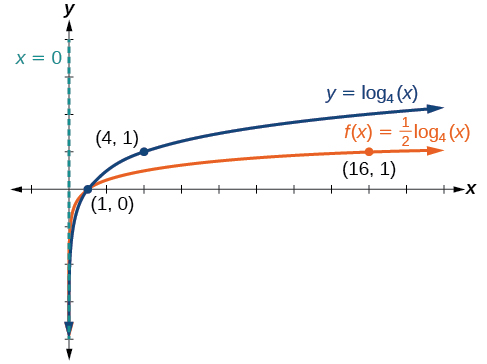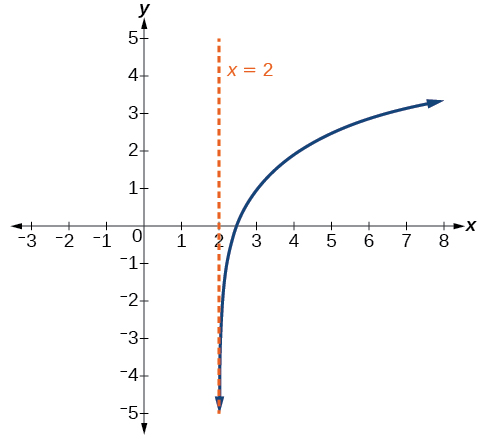# 6.4 Graphs of logarithmic functions  (Page 5/8)

 Page 5 / 8

Given a logarithmic function with the form $\text{\hspace{0.17em}}f\left(x\right)=a{\mathrm{log}}_{b}\left(x\right),$ $a>0,$ graph the translation.

1. Identify the vertical stretch or compressions:
• If $\text{\hspace{0.17em}}|a|>1,$ the graph of $\text{\hspace{0.17em}}f\left(x\right)={\mathrm{log}}_{b}\left(x\right)\text{\hspace{0.17em}}$ is stretched by a factor of $\text{\hspace{0.17em}}a\text{\hspace{0.17em}}$ units.
• If $\text{\hspace{0.17em}}|a|<1,$ the graph of $\text{\hspace{0.17em}}f\left(x\right)={\mathrm{log}}_{b}\left(x\right)\text{\hspace{0.17em}}$ is compressed by a factor of $\text{\hspace{0.17em}}a\text{\hspace{0.17em}}$ units.
2. Draw the vertical asymptote $\text{\hspace{0.17em}}x=0.$
3. Identify three key points from the parent function. Find new coordinates for the shifted functions by multiplying the $\text{\hspace{0.17em}}y\text{\hspace{0.17em}}$ coordinates by $\text{\hspace{0.17em}}a.$
4. Label the three points.
5. The domain is $\text{\hspace{0.17em}}\left(0,\infty \right),$ the range is $\text{\hspace{0.17em}}\left(-\infty ,\infty \right),$ and the vertical asymptote is $\text{\hspace{0.17em}}x=0.$

## Graphing a stretch or compression of the parent function y = log b ( x )

Sketch a graph of $\text{\hspace{0.17em}}f\left(x\right)=2{\mathrm{log}}_{4}\left(x\right)\text{\hspace{0.17em}}$ alongside its parent function. Include the key points and asymptote on the graph. State the domain, range, and asymptote.

Since the function is $\text{\hspace{0.17em}}f\left(x\right)=2{\mathrm{log}}_{4}\left(x\right),$ we will notice $\text{\hspace{0.17em}}a=2.$

This means we will stretch the function $\text{\hspace{0.17em}}f\left(x\right)={\mathrm{log}}_{4}\left(x\right)\text{\hspace{0.17em}}$ by a factor of 2.

The vertical asymptote is $\text{\hspace{0.17em}}x=0.$

Consider the three key points from the parent function, $\text{\hspace{0.17em}}\left(\frac{1}{4},-1\right),$ $\left(1,0\right),\text{\hspace{0.17em}}$ and $\text{\hspace{0.17em}}\left(4,1\right).$

The new coordinates are found by multiplying the $\text{\hspace{0.17em}}y\text{\hspace{0.17em}}$ coordinates by 2.

Label the points $\text{\hspace{0.17em}}\left(\frac{1}{4},-2\right),$ $\left(1,0\right)\text{\hspace{0.17em}},$ and $\text{\hspace{0.17em}}\left(4,\text{2}\right).$

The domain is $\text{\hspace{0.17em}}\left(0,\text{\hspace{0.17em}}\infty \right),$ the range is $\text{\hspace{0.17em}}\left(-\infty ,\infty \right),\text{\hspace{0.17em}}$ and the vertical asymptote is $\text{\hspace{0.17em}}x=0.\text{\hspace{0.17em}}$ See [link] .

The domain is $\text{\hspace{0.17em}}\left(0,\infty \right),$ the range is $\text{\hspace{0.17em}}\left(-\infty ,\infty \right),$ and the vertical asymptote is $\text{\hspace{0.17em}}x=0.$

Sketch a graph of $\text{\hspace{0.17em}}f\left(x\right)=\frac{1}{2}\text{\hspace{0.17em}}{\mathrm{log}}_{4}\left(x\right)\text{\hspace{0.17em}}$ alongside its parent function. Include the key points and asymptote on the graph. State the domain, range, and asymptote.The domain is $\text{\hspace{0.17em}}\left(0,\infty \right),$ the range is $\text{\hspace{0.17em}}\left(-\infty ,\infty \right),$ and the vertical asymptote is $\text{\hspace{0.17em}}x=0.$

## Combining a shift and a stretch

Sketch a graph of $\text{\hspace{0.17em}}f\left(x\right)=5\mathrm{log}\left(x+2\right).\text{\hspace{0.17em}}$ State the domain, range, and asymptote.

Remember: what happens inside parentheses happens first. First, we move the graph left 2 units, then stretch the function vertically by a factor of 5, as in [link] . The vertical asymptote will be shifted to $\text{\hspace{0.17em}}x=-2.\text{\hspace{0.17em}}$ The x -intercept will be $\text{\hspace{0.17em}}\left(-1,0\right).\text{\hspace{0.17em}}$ The domain will be $\text{\hspace{0.17em}}\left(-2,\infty \right).\text{\hspace{0.17em}}$ Two points will help give the shape of the graph: $\text{\hspace{0.17em}}\left(-1,0\right)\text{\hspace{0.17em}}$ and $\text{\hspace{0.17em}}\left(8,5\right).\text{\hspace{0.17em}}$ We chose $\text{\hspace{0.17em}}x=8\text{\hspace{0.17em}}$ as the x -coordinate of one point to graph because when $\text{\hspace{0.17em}}x=8,\text{\hspace{0.17em}}$ $\text{\hspace{0.17em}}x+2=10,\text{\hspace{0.17em}}$ the base of the common logarithm.

The domain is $\text{\hspace{0.17em}}\left(-2,\infty \right),$ the range is $\text{\hspace{0.17em}}\left(-\infty ,\infty \right),$ and the vertical asymptote is $\text{\hspace{0.17em}}x=-2.$

Sketch a graph of the function $\text{\hspace{0.17em}}f\left(x\right)=3\mathrm{log}\left(x-2\right)+1.\text{\hspace{0.17em}}$ State the domain, range, and asymptote.The domain is $\text{\hspace{0.17em}}\left(2,\infty \right),$ the range is $\text{\hspace{0.17em}}\left(-\infty ,\infty \right),$ and the vertical asymptote is $\text{\hspace{0.17em}}x=2.$

## Graphing reflections of f ( x ) = log b ( x )

When the parent function $\text{\hspace{0.17em}}f\left(x\right)={\mathrm{log}}_{b}\left(x\right)\text{\hspace{0.17em}}$ is multiplied by $\text{\hspace{0.17em}}-1,$ the result is a reflection about the x -axis. When the input is multiplied by $\text{\hspace{0.17em}}-1,$ the result is a reflection about the y -axis. To visualize reflections, we restrict $\text{\hspace{0.17em}}b>1,\text{\hspace{0.17em}}$ and observe the general graph of the parent function $\text{\hspace{0.17em}}f\left(x\right)={\mathrm{log}}_{b}\left(x\right)\text{\hspace{0.17em}}$ alongside the reflection about the x -axis, $\text{\hspace{0.17em}}g\left(x\right)={\mathrm{-log}}_{b}\left(x\right)\text{\hspace{0.17em}}$ and the reflection about the y -axis, $\text{\hspace{0.17em}}h\left(x\right)={\mathrm{log}}_{b}\left(-x\right).$

## Reflections of the parent function y = log b ( x )

The function $\text{\hspace{0.17em}}f\left(x\right)={\mathrm{-log}}_{b}\left(x\right)$

• reflects the parent function $\text{\hspace{0.17em}}y={\mathrm{log}}_{b}\left(x\right)\text{\hspace{0.17em}}$ about the x -axis.
• has domain, $\text{\hspace{0.17em}}\left(0,\infty \right),$ range, $\text{\hspace{0.17em}}\left(-\infty ,\infty \right),$ and vertical asymptote, $\text{\hspace{0.17em}}x=0,$ which are unchanged from the parent function.

The function $\text{\hspace{0.17em}}f\left(x\right)={\mathrm{log}}_{b}\left(-x\right)$

• reflects the parent function $\text{\hspace{0.17em}}y={\mathrm{log}}_{b}\left(x\right)\text{\hspace{0.17em}}$ about the y -axis.
• has domain $\text{\hspace{0.17em}}\left(-\infty ,0\right).$
• has range, $\text{\hspace{0.17em}}\left(-\infty ,\infty \right),$ and vertical asymptote, $\text{\hspace{0.17em}}x=0,$ which are unchanged from the parent function.

bsc F. y algebra and trigonometry pepper 2
given that x= 3/5 find sin 3x
4
DB
remove any signs and collect terms of -2(8a-3b-c)
-16a+6b+2c
Will
Joeval
(x2-2x+8)-4(x2-3x+5)
sorry
Miranda
x²-2x+9-4x²+12x-20 -3x²+10x+11
Miranda
x²-2x+9-4x²+12x-20 -3x²+10x+11
Miranda
(X2-2X+8)-4(X2-3X+5)=0 ?
master
The anwser is imaginary number if you want to know The anwser of the expression you must arrange The expression and use quadratic formula To find the answer
master
The anwser is imaginary number if you want to know The anwser of the expression you must arrange The expression and use quadratic formula To find the answer
master
Y
master
master
Soo sorry (5±Root11* i)/3
master
Mukhtar
explain and give four example of hyperbolic function
What is the correct rational algebraic expression of the given "a fraction whose denominator is 10 more than the numerator y?
y/y+10
Mr
Find nth derivative of eax sin (bx + c).
Find area common to the parabola y2 = 4ax and x2 = 4ay.
Anurag
A rectangular garden is 25ft wide. if its area is 1125ft, what is the length of the garden
to find the length I divide the area by the wide wich means 1125ft/25ft=45
Miranda
thanks
Jhovie
What do you call a relation where each element in the domain is related to only one value in the range by some rules?
A banana.
Yaona
given 4cot thither +3=0and 0°<thither <180° use a sketch to determine the value of the following a)cos thither
what are you up to?
nothing up todat yet
Miranda
hi
jai
hello
jai
Miranda Drice
jai
aap konsi country se ho
jai
which language is that
Miranda
I am living in india
jai
good
Miranda
what is the formula for calculating algebraic
I think the formula for calculating algebraic is the statement of the equality of two expression stimulate by a set of addition, multiplication, soustraction, division, raising to a power and extraction of Root. U believe by having those in the equation you will be in measure to calculate it
Miranda
state and prove Cayley hamilton therom
hello
Propessor
hi
Miranda
the Cayley hamilton Theorem state if A is a square matrix and if f(x) is its characterics polynomial then f(x)=0 in another ways evey square matrix is a root of its chatacteristics polynomial.
Miranda
hi
jai
hi Miranda
jai
thanks
Propessor
welcome
jai
What is algebra
algebra is a branch of the mathematics to calculate expressions follow.
Miranda
Miranda Drice would you mind teaching me mathematics? I think you are really good at math. I'm not good at it. In fact I hate it. 😅😅😅
Jeffrey
lolll who told you I'm good at it
Miranda
something seems to wispher me to my ear that u are good at it. lol
Jeffrey
lolllll if you say so
Miranda
but seriously, Im really bad at math. And I hate it. But you see, I downloaded this app two months ago hoping to master it.
Jeffrey
which grade are you in though
Miranda
oh woww I understand
Miranda
Jeffrey
Jeffrey
Miranda
how come you finished in college and you don't like math though
Miranda
gotta practice, holmie
Steve
if you never use it you won't be able to appreciate it
Steve
I don't know why. But Im trying to like it.
Jeffrey
yes steve. you're right
Jeffrey
so you better
Miranda
what is the solution of the given equation?
which equation
Miranda
I dont know. lol
Jeffrey
Miranda
JeffreyByByBy OpenStaxBy OpenStaxBy Keyaira BraxtonBy Rachel CarlisleBy Anonymous UserBy OpenStaxBy OpenStaxBy Cath YuBy Brooke DelaneyBy Sarah Warren